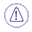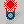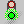# Keywords

 if...else ... else... if let for inside while setWhen working with the boolean value parameters `true` and `false`, make sure to use these values in English. Do not use the equivalent of true and false in your own language.

## if...else ... else...if

#### Rules

if  ... else  ... else if
Conditionally executes a group of statements, depending on the value of an expression. You can use either block form syntaxes:
 ``if`` condition statements [else elsestatements ]`` `or` ````if```` condition      { statements } [else if condition-n       [  { elseifstatements  } ] ] . . . [else     [  { elsestatements } ] ]``````
You can use the single-line form (first syntax) for short, simple rules. However, the block form (second syntax) provides more structure and flexibility than the single-line form and is usually easier to read, maintain, and test.
The else and else if clauses are both optional. You can have as many else if statements as you want below a block if, but none can appear after the else clause. Block if statements can be nested that is, contained within one another.

#### Checks

Checks can only read parameters. As a consequence, you cannot use functions that have arguments in output.

 ``Relations\Formula.1\Activity == false``

Checks can use a specific keyword =>. statement1  => statement2  (if statement1  then statement2).
Displays a message (if type is Warning or Information) and turns to red in the specification tree each time statement2 is invalid as statement1 is fulfilled.

 OK => KOKO => KOKO => OKOK => OK## For Statement

Note that the For statement is available for Action and Reaction scripts only.

 The first usage of the For keyword is a loop based on the element of a list. See syntax opposite.Where: x is a variable name (of a given type. It may represent an object or a value). x can be used in the body (like any other variable of the language). It contains the element of the list corresponding to the current iteration. List is a variable name of type List or an expression returning a list. The body is executed Nth times where N is the number of elements of the list. ```et List.1(List) let x(Point) For x inside List { … Body … if (x <> NULL) }``` The second usage of the For keyword executes a loop until an expression becomes false. See syntax opposite.Where: x is a variable name of integer type. It is incremented at the end of each execution of the body. predicate is a Boolean expression. The body is executed if this expression is true. This expression is evaluated before the body. Note that the second usage of the For operator can lead to infinite loops. ```For x while predicate { … Body … }```

## While Statement

 This loop executes until an expression becomes false. See syntax opposite.Where: i is a variable name of integer type. It is incremented at the end of each execution of the body. X is a variable for points. ```let i = 1 let x(Point) for i while i<=parameter.Size() { x = parameter.GetItem(i) if (x.GetAttributeReal("Y") < 0.04) x.SetAttributeReal("Y",0.04) }```

## let

Temporary variables can be declared at the beginning of the script by using the let keyword. A temporary variable does not persist as a parameter after the rule execution is finished.

 ``````/*Rule created by CRE 08/23/99*/ let x = 5 mm if PartBody\Hole.1\Diameter > x { PartBody\Hole.1\Activity = false } ```` ````

For non digital values, the type has to be indicated:

```let S(Surface) S= split (...,...)```

Temporary variables must be declared at the beginning of the rule, before any conditional instruction is specified.

 ```let S1(Surface) let S2(Surface) let S3(Surface) ``` ```S1 = Split ... S2 = ... S3 = ...```

## set

If you want to manipulate a type, you can use the set keyword to set a variable of this type and type directly the attribute to read and/or write its characteristics.

 ``````/*You can start from an object in a variable x and want to manipulate it as a Hole. To do so, use the set keyword to indicate that you want to manipulate the object as a Hole*/ let y(Hole) set y = x if (y <> NULL) Message ("Hole diameter is #",y.Diameter)``````